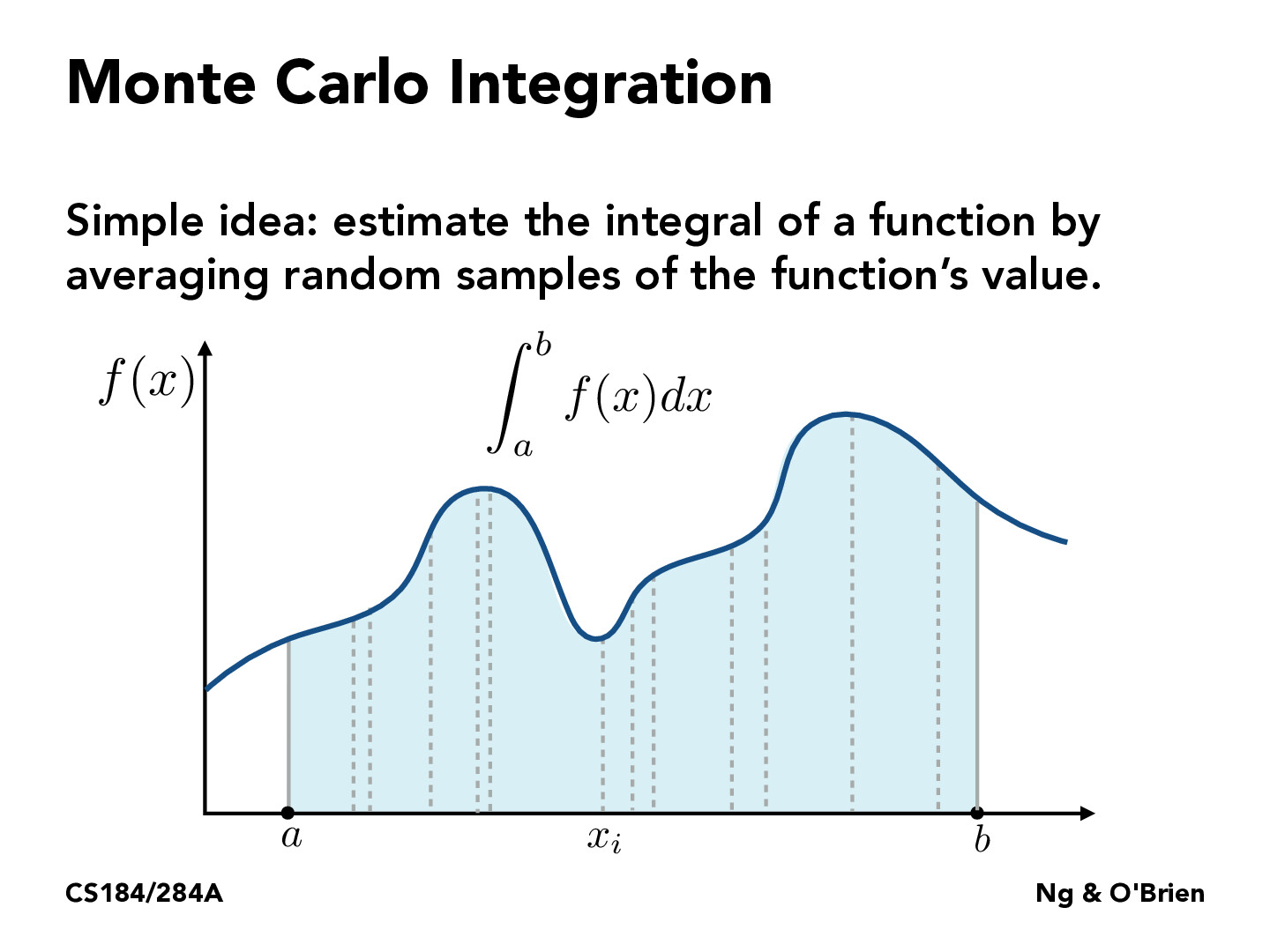Lecture 12: Monte Carlo Integration (20)lwg0320

What is the advantage of taking random samples?Sicheng-Pan

Averaging random samples may help when it is hard or inefficient to derive symbolic answer for the integration. This method is also easy to parallelize.rsha256

@Sicheng-Pan all of those things can be achieved by a uniform np.linspace spaced samples. Why specifically do we make it stochastic? Is it for speedup purposes?andrewhuang56

One benefit of sampling stochastically is that it's a tad bit more reliable in a general case. Let's say that we wanted to estimate $\int_0^{n} \cos (2\pi x)\, dx$, and sampled at intervals of $1.$ Then, whenever we sampled, we would get $\cos (2i\pi) = 1$. Even if we adjusted the integral a bit: $\int_0^{n} \cos ((2\pi+\varepsilon) x)\, dx,$ for small-ish $n$, we would get the same problem. Of course, this is a "worst case", and changing this to, say intervals of $\frac{1}{2},$ would give us a much much better answer. However, if we sample stochastically, there isn't really a "worst case" at all. Of course, we might have to sample more, but there is no "trial and error" (of, say, the sample size) necessary to get a good estimate for the integral. Especially for more complicated functions, maybe it's the case that it one cannot simply inspect a good interval length.ShrihanSolo

I would imagine MC integration to perform badly for highly stochastic functions. For example, a mostly uniform function with small spikes is likely to produce a bad estimate using MC integration.

You must be enrolled in the course to comment